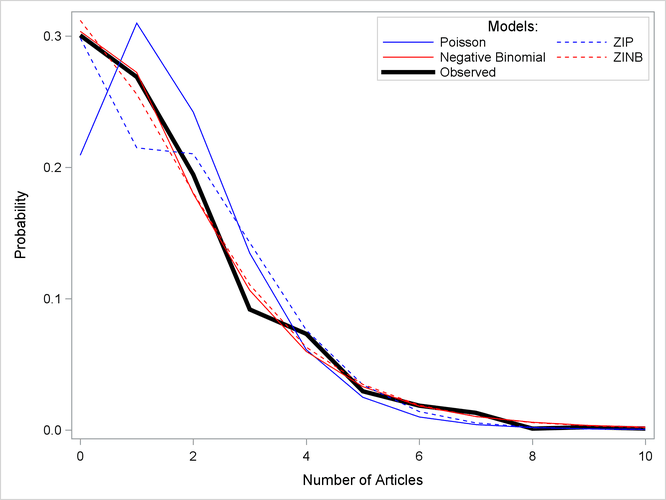The COUNTREG Procedure

Example 11.2 ZIP and ZINB Models for Data That Exhibit Extra Zeros

In the study by Long (1997) of the number of published articles by scientists (see the section Getting Started: COUNTREG Procedure), the observed proportion of scientists who publish no articles is 0.3005. The following statements use PROC FREQ to compute the proportion of scientists who publish each observed number of articles. Output 11.2.1 shows the results.

proc freq data=long97data;
table art / out=obs;
run;


Output 11.2.1: Proportion of Scientists Who Publish a Certain Number of Articles

The FREQ Procedure

art Frequency Percent Cumulative
Frequency
Cumulative
Percent
0 275 30.05 275 30.05
1 246 26.89 521 56.94
2 178 19.45 699 76.39
3 84 9.18 783 85.57
4 67 7.32 850 92.90
5 27 2.95 877 95.85
6 17 1.86 894 97.70
7 12 1.31 906 99.02
8 1 0.11 907 99.13
9 2 0.22 909 99.34
10 1 0.11 910 99.45
11 1 0.11 911 99.56
12 2 0.22 913 99.78
16 1 0.11 914 99.89
19 1 0.11 915 100.00

PROC COUNTREG is then used to fit Poisson and negative binomial models to the data. For each model, the PROBCOUNT option computes the probability that the number of published articles is m, for m = 0 to 10. The following statements compute the estimates for Poisson and negative binomial models. The MEAN procedure is then used to compute the average probability of a zero response.

proc countreg data=long97data;
model art=fem mar kid5 phd ment / dist=poisson;
output out=predpoi probcount(0 to 10);
run;

proc means mean data=predpoi;
var p_0;
run;


The output from the Poisson model for the COUNTREG and MEAN procedures is shown in Output 11.2.2.

Output 11.2.2: Poisson Model Estimation

The COUNTREG Procedure

Model Fit Summary
Dependent Variable art
Number of Observations 915
Data Set WORK.LONG97DATA
Model Poisson
Log Likelihood -1651
Number of Iterations 5
Optimization Method Newton-Raphson
AIC 3314
SBC 3343

 Algorithm converged.

Parameter Estimates
Parameter DF Estimate Standard
Error
t Value Approx
Pr > |t|
Intercept 1 0.304617 0.102982 2.96 0.0031
fem 1 -0.224594 0.054614 -4.11 <.0001
mar 1 0.155243 0.061375 2.53 0.0114
kid5 1 -0.184883 0.040127 -4.61 <.0001
phd 1 0.012823 0.026397 0.49 0.6271
ment 1 0.025543 0.002006 12.73 <.0001

The MEANS Procedure

Analysis Variable
: P_0 Probability
of art taking level=0
Mean
0.2092071

The following statements show the syntax for the negative binomial model:

proc countreg data=long97data plots(only)=profilelike;
model art=fem mar kid5 phd ment / dist=negbin(p=2) method=qn;
output out=prednb probcount(0 to 10);
run;

proc means mean data=prednb;
var p_0;
run;


Output 11.2.3: Profile Likelihood Functions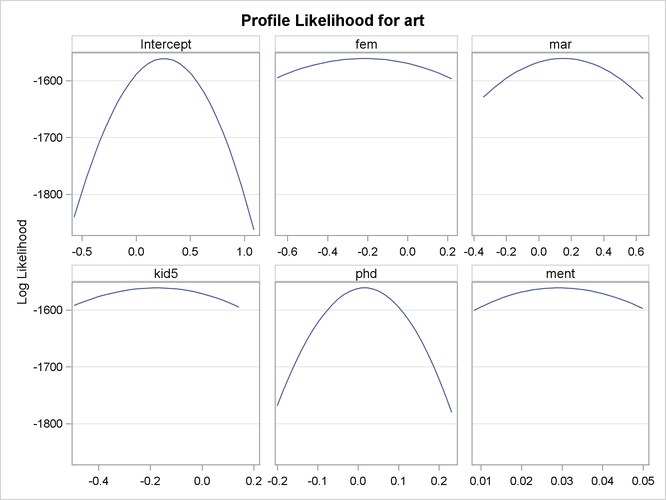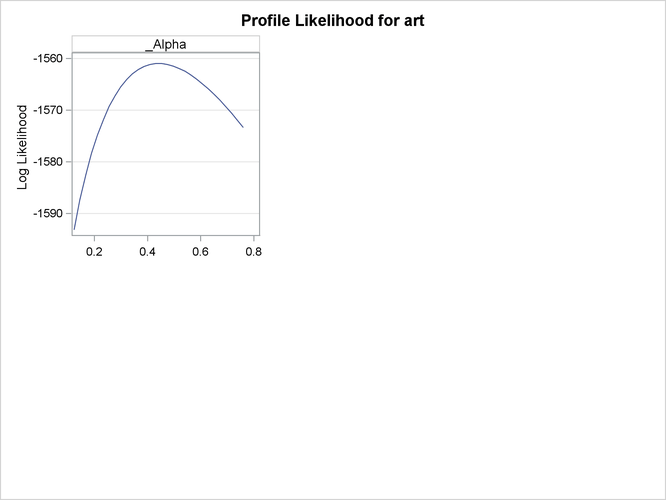Output 11.2.3 show the profile likelihood functions of the negative binomial model for the Long (1997) data set, in which each model parameter is varied while holding all others fixed at the MLE. This can serve as a diagnostic tool for model performance, because a large number of flat profile likelihood functions indicates poor optimization results and the resulting MLE should be used with caution.

Output 11.2.4 shows the results of the preceding statements.

Output 11.2.4: Negative Binomial Model Estimation

The COUNTREG Procedure

Model Fit Summary
Dependent Variable art
Number of Observations 915
Data Set WORK.LONG97DATA
Model NegBin(p=2)
Log Likelihood -1561
Number of Iterations 16
Optimization Method Quasi-Newton
AIC 3136
SBC 3170

 Algorithm converged.

Parameter Estimates
Parameter DF Estimate Standard
Error
t Value Approx
Pr > |t|
Intercept 1 0.256144 0.138560 1.85 0.0645
fem 1 -0.216418 0.072672 -2.98 0.0029
mar 1 0.150489 0.082106 1.83 0.0668
kid5 1 -0.176415 0.053060 -3.32 0.0009
phd 1 0.015271 0.036040 0.42 0.6718
ment 1 0.029082 0.003470 8.38 <.0001
_Alpha 1 0.441620 0.052967 8.34 <.0001

The MEANS Procedure

Analysis Variable
: P_0 Probability
of art taking level=0
Mean
0.3035957

For each model, the predicted proportion of zero articles can be calculated as the average predicted probability of zero articles across all scientists. Under the Poisson model, the predicted proportion of zero articles is 0.2092, which considerably underestimates the observed proportion. The negative binomial more closely estimates the proportion of zeros (0.3036). Also, the test of the dispersion parameter, _Alpha, in the negative binomial model indicates significant overdispersion (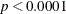). As a result, the negative binomial model is preferred to the Poisson model.

Another way to account for the large number of zeros in this data set is to fit a zero-inflated Poisson (ZIP) or a zero-inflated negative binomial (ZINB) model. In the following statements, DIST=ZIP requests the ZIP model. In the ZEROMODEL statement, you can specify the predictors,, for the process that generates the additional zeros. The ZEROMODEL statement also specifies the model for the probability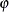. By default, a logistic model is used for. You can change the default by using the LINK= option. In this particular ZIP model, all variables that are used to model the article counts are also used to model.

proc countreg data=long97data;
model art = fem mar kid5 phd ment / dist=zip;
zeromodel art ~ fem mar kid5 phd ment;
output out=predzip probcount(0 to 10);
run;

proc means data=predzip mean;
var p_0;
run;


The parameters of the ZIP model are displayed in Output 11.2.5. The first set of parameters gives the estimates ofin the model for the Poisson process mean. Parameters that have the prefix "Inf_" are the estimates of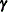in the logistic model for.

Output 11.2.5: ZIP Model Estimation

The COUNTREG Procedure

Model Fit Summary
Dependent Variable art
Number of Observations 915
Data Set WORK.LONG97DATA
Model ZIP
Log Likelihood -1605
Number of Iterations 16
Optimization Method Newton-Raphson
AIC 3234
SBC 3291

 Algorithm converged.

Parameter Estimates
Parameter DF Estimate Standard
Error
t Value Approx
Pr > |t|
Intercept 1 0.640838 0.121306 5.28 <.0001
fem 1 -0.209145 0.063405 -3.30 0.0010
mar 1 0.103751 0.071111 1.46 0.1446
kid5 1 -0.143320 0.047429 -3.02 0.0025
phd 1 -0.006166 0.031008 -0.20 0.8424
ment 1 0.018098 0.002295 7.89 <.0001
Inf_Intercept 1 -0.577060 0.509383 -1.13 0.2573
Inf_fem 1 0.109747 0.280082 0.39 0.6952
Inf_mar 1 -0.354013 0.317611 -1.11 0.2650
Inf_kid5 1 0.217101 0.196481 1.10 0.2692
Inf_phd 1 0.001272 0.145262 0.01 0.9930
Inf_ment 1 -0.134114 0.045244 -2.96 0.0030

The MEANS Procedure

Analysis Variable
: P_0 Probability
of art taking level=0
Mean
0.2985679

The proportion of zeros that are predicted by the ZIP model is 0.2986, which is much closer to the observed proportion than the Poisson model. But Output 11.2.7 shows that both models deviate from the observed proportions at one, two, and three articles.

The ZINB model is specified by the DIST=ZINB option. All variables are again used to model both the number of articles and. The METHOD=QN option specifies that the quasi-Newton method be used to fit the model rather than the default Newton-Raphson method. These options are implemented in the following statements:

proc countreg data=long97data;
model art=fem mar kid5 phd ment / dist=zinb method=qn;
zeromodel art ~ fem mar kid5 phd ment;
output out=predzinb probcount(0 to 10);
run;

proc means data=predzinb mean;
var p_0;
run;


The estimated parameters of the ZINB model are shown in Output 11.2.6. The test for overdispersion again indicates a preference for the negative binomial version of the zero-inflated model (). The ZINB model also does a good job of estimating the proportion of zeros (0.3119), and it follows the observed proportions well, though possibly not as well as the negative binomial model.

Output 11.2.6: ZINB Model Estimation

The COUNTREG Procedure

Model Fit Summary
Dependent Variable art
Number of Observations 915
Data Set WORK.LONG97DATA
Model ZINB
Log Likelihood -1550
Number of Iterations 84
Optimization Method Quasi-Newton
AIC 3126
SBC 3189

 Algorithm converged.

Parameter Estimates
Parameter DF Estimate Standard
Error
t Value Approx
Pr > |t|
Intercept 1 0.416747 0.143596 2.90 0.0037
fem 1 -0.195507 0.075592 -2.59 0.0097
mar 1 0.097583 0.084452 1.16 0.2479
kid5 1 -0.151732 0.054206 -2.80 0.0051
phd 1 -0.000700 0.036270 -0.02 0.9846
ment 1 0.024786 0.003493 7.10 <.0001
Inf_Intercept 1 -0.191685 1.322809 -0.14 0.8848
Inf_fem 1 0.635932 0.848913 0.75 0.4538
Inf_mar 1 -1.499469 0.938661 -1.60 0.1102
Inf_kid5 1 0.628427 0.442780 1.42 0.1558
Inf_phd 1 -0.037715 0.308005 -0.12 0.9025
Inf_ment 1 -0.882293 0.316224 -2.79 0.0053
_Alpha 1 0.376681 0.051029 7.38 <.0001

The MEANS Procedure

Analysis Variable
: P_0 Probability
of art taking level=0
Mean
0.3119487

The following statements compute the average predicted count probability across all scientists for each count 0, 1,, 10. The averages for each model, along with the observed proportions, are then arranged for plotting by PROC SGPLOT.

proc summary data=predpoi;
var p_0-p_10;
output out=mnpoi mean(p_0-p_10)=mn0-mn10;
run;
proc summary data=prednb;
var p_0-p_10;
output out=mnnb mean(p_0-p_10)=mn0-mn10;
run;
proc summary data=predzip;
var p_0-p_10;
output out=mnzip mean(p_0-p_10)=mn0-mn10;
run;
proc summary data=predzinb;
var p_0-p_10;
output out=mnzinb mean(p_0-p_10)=mn0-mn10;
run;

data means;
set mnpoi mnnb mnzip mnzinb;
drop _type_ _freq_;
run;

proc transpose data=means out=tmeans;
run;

data allpred;
merge obs(where=(art<=10)) tmeans;
obs=percent/100;
run;

proc sgplot;
yaxis label='Probability';
xaxis label='Number of Articles';
series y=obs  x=art / name='obs' legendlabel='Observed'
lineattrs=(color=black thickness=4px);
series y=col1 x=art / name='poi' legendlabel='Poisson'
lineattrs=(color=blue);
series y=col2 x=art/ name='nb' legendlabel='Negative Binomial'
lineattrs=(color=red);
series y=col3 x=art/ name='zip' legendlabel='ZIP'
lineattrs=(color=blue pattern=2);
series y=col4 x=art/ name='zinb' legendlabel='ZINB'
lineattrs=(color=red pattern=2);
discretelegend 'poi' 'zip' 'nb' 'zinb' 'obs' / title='Models:'
location=inside position=ne across=2 down=3;
run;


For each of the four fitted models, Output 11.2.7 shows the average predicted count probability for each article count across all scientists. The Poisson model clearly underestimates the proportion of zero articles published, whereas the other three models are quite accurate at zero. All the models do well at the larger numbers of articles.

Output 11.2.7: Average Predicted Count Probability